## (a) How long in seconds does it take a radio signal to travel 160 km from a transmitter to a receiving antenna? (b) We see a full Moon by re

Question

(a) How long in seconds does it take a radio signal to travel 160 km from a transmitter to a receiving antenna? (b) We see a full Moon by reflected sunlight. How much earlier did the light that enters our eye leave the Sun? The Earth – Moon and Earth – Sun distances are 3.8×105 km and 1.5 × 108 km, respectively. (c) What is the round-trip travel time in seconds for light between Earth and a spaceship at a 3.6 × 108 km distance from Earth? (d) Suppose astronomers observe a supernova about 9300 light-years (ly) distant. How long ago in years did the explosion actually occur?

in progress 0
2 months 2021-08-02T19:22:55+00:00 1 Answers 2 views 0

1. Explanation:

Given:

Distance between the transmitter and receiver = 160 km

Distance between Earth and Sun (D) = 150000000 km

Distance between Earth and Moon (D’) = 380000 km

Distance between Earth and space ship (D”) = 360000000 km

Distance between Earth and Supernova (d) = 9300 LY

We know that,

Speed of Light = 300000 km/sec

Lets us solve each problem now:

a) We know that the radio waves travle with speed of light. So the time(T) taken by the signal from transmitter to receiving antenna will be,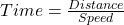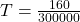T = 0.53 millisecond

b) Time taken by sunlight to reach Earth is,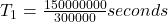T₁ = 500 seconds = 08 min 20 seconds

Time taken by light from Moon to reach Earth will be,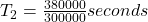T₂ = 1.26 seconds

Let us assume that in the given situation Earth and Moon are at the same distance from the Sun. This means that Sun light will take the same time to reach Moon as it will take to reach Earth. So Sun light reaches the Moon in 500 seconds. After that it will be reflected towards Earth and will take 1.26 seconds more to reach Earth.

Thus the light left the Sun 501.26 seconds earlier.

c) Time taken by the light from space ship to reach Earth will be,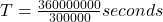T = 1200 seconds.

Thus round trip time will be = 2T = 2400 seconds

d) Lightyear is a unit of distance and is equal to the distance travelled by light in a year. If a supernova is at a distance of 9300 LY it means that light has taken 9300 years to reach Earth from that supernova. It means that the light we see today was actually generated from the supernova 9300 years ago.

It is evident that the explosion actually occurred 9300 years back.